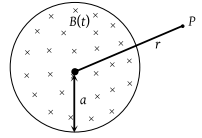• Subject:

...

• Topic:

...

A uniform but time-varying magnetic field B(t) exists in a circular region of radius a and is directed into the plane of the paper, as shown. The magnitude of the induced electric field at point P at a distance r from the centre of the circular region(1) Is zero

(2) Decreases as $\frac{1}{r}$

(3) Increases as r

(4) Decreases as $\frac{1}{{r}^{2}}$